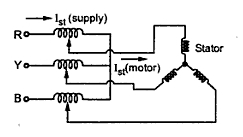### Autotransformer Starter

A three phase star connected autotransformer can be used to reduce the voltage applied to the stator. Such a starter is called an autotransformer starter. The schematic diagram of autotransformer starter. The schematic diagram of autotransformer starter is shown in the Fig..1.Fig. 1  Autotransformer starter

It consists of a suitable change over switch.
When the switch is in the start position, the stator winding is supplied with reduced voltage. This can be controlled by tappings provided with autotransformer.
The reduction in applied voltage by the fractional percentage tappings x, used for an autotransformer is shown in the Fig. 2.Fig.2  Use of auotransformer to reduce voltage at start

When motor gathers 80% of the normal speed, the change over switch is thrown into run position.
Due to this, rated voltage gets applied to stator winding. The motor starts rotating with normal speed. Changing of switch is done automatically by using relays. The power loss is much less in this type of starting. It can be used for both star and delta connected motors. But it is expensive than stator resistance starter.
1.1 Relation between Tst  and TF.L.
Let x be the fractional percentage tappings used for an autotransformer to apply reduced voltage to the stator.
So if,           Isc = Starting motor current at rated voltage
and              Ist  = Starting motor current with starter
then             Ist = x Isc    .....Motor side              ............(1)
But there is exists a fixed ratio between starting current drawn from supply Ist (supply) and starting moor current Ist (motor) due to autotransformer, as shown in the Fig.3.Fig. 3
Autotransformer ratio x = Ist (supply)/ Ist (motor)
Ist (supply) = x Ist (motor)                                  .............(2)
Substituting Ist (motor) from equation (1),
...     Ist (supply) = x . x Isc = x2 Isc                              ............(3)
Now Tst  α  Ist2 (motor) α x2 Isc2
and  TF.L.  α  (IF.L.)2/sf

Note : Thus starting torque reduces by x2 where x is the transformer ratio.
Example :  A squirrel cage induction motor has a full load slip of 5%. The motor starting current at rated voltage is 6 times its full load current. Find the tapping on the autotransformer starter which would give full load torque at start. Whet would then be the supply starting current ?
Solution : Starting current at rated voltage = Isc
...           Isc = 6 IF.L.    and sf = 5% = 0.05
Let    x = Tapping on autotransformer
TF.L.  = Tst

1 = x2 (6/1)2 x 0.05
x = 0.7453
Thus 74.53% tapping is required
Now Ist (supply) = x Ist (motor) = x (xIsc) = x2 Isc
= x2 x 6 = 3.33 IF.L.
Thus supply starting current is 3.33 times the full load current.